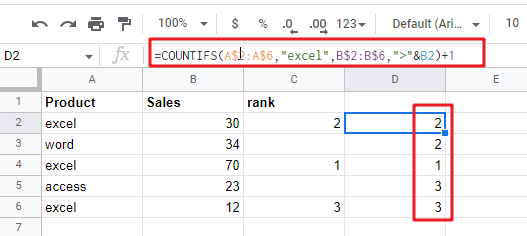# Rank Based on Specific Value in google sheets

This article will guide you how to sort the values in a given column based on a specific value in another column in google sheets. How to sort a range of values in google sheets by using IF function and COUNTIFS function based on a specific value in another column.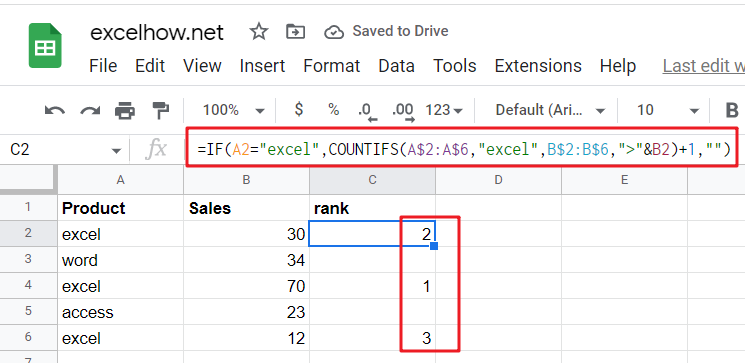## Rank Based on Specific Value

Suppose you have a list with two columns of data, where the first column is a list of products and another column is the number of sales. If you have an urgent need to quickly sort the number of sales for a given product name, you can’t solve this request with the RANK function, because it can only be used to sort values up to one range. So is there a quick and easy way for sorting in google sheets? Of course yes, the following will show how to use the IF function in combination with COUNTIFS function to build a new google sheets formula to solve the problem. The formula is as follows.

For example, if you want to rank the sales values in the range B2:B6 based on the specified product name “excel” in the range A2:A6, then you can use the following formula to get the ranking results：

`=IF(A2="excel",COUNTIFS(A\$2:A\$6,"excel",B\$2:B\$6,">"&B2)+1,"")`

Next, you need to type the above formula in another blank cell and press Enter on your keyboard to apply the formula, and after that you need to drag the autofill handler down to the other cells to apply this formula.As you can see from the example above, the products in column A, “excel“, can now be ranked by their sales.

## Let’s See How This Formula Works

### =COUNTIFS(A\$2:A\$6,”excel”)

This formula is used to count the number of excel product names in a given cell range A2:A6.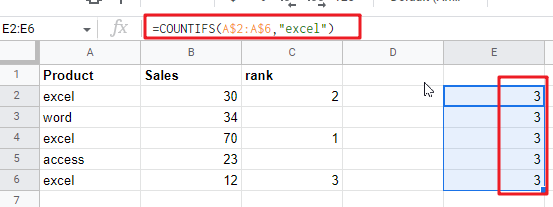### =COUNTIFS(B\$2:B\$6,”>”&B2)

This formula is used to count the number of times that the value in the given cell range B2:B6 is greater than the current sales value.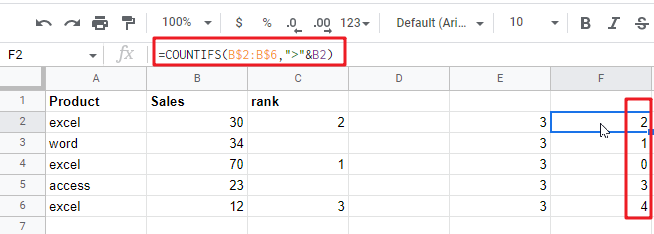### =COUNTIFS(A\$2:A\$6,”excel”,B\$2:B\$6,”>”&B2)

The formula is used to count the number of products with sales greater than the current product sales, and must meet one additional condition is that the product name must be excel.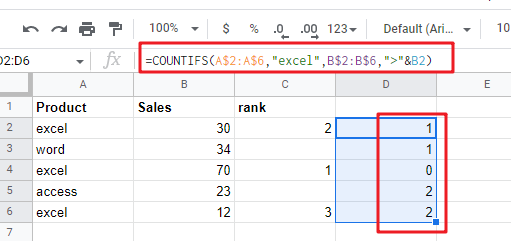### =COUNTIFS(A\$2:A\$6,”excel”,B\$2:B\$6,”>”&B2)+1

The returned result of the above formula adding the number 1 is the actual ranking of the specified product in its own product list.